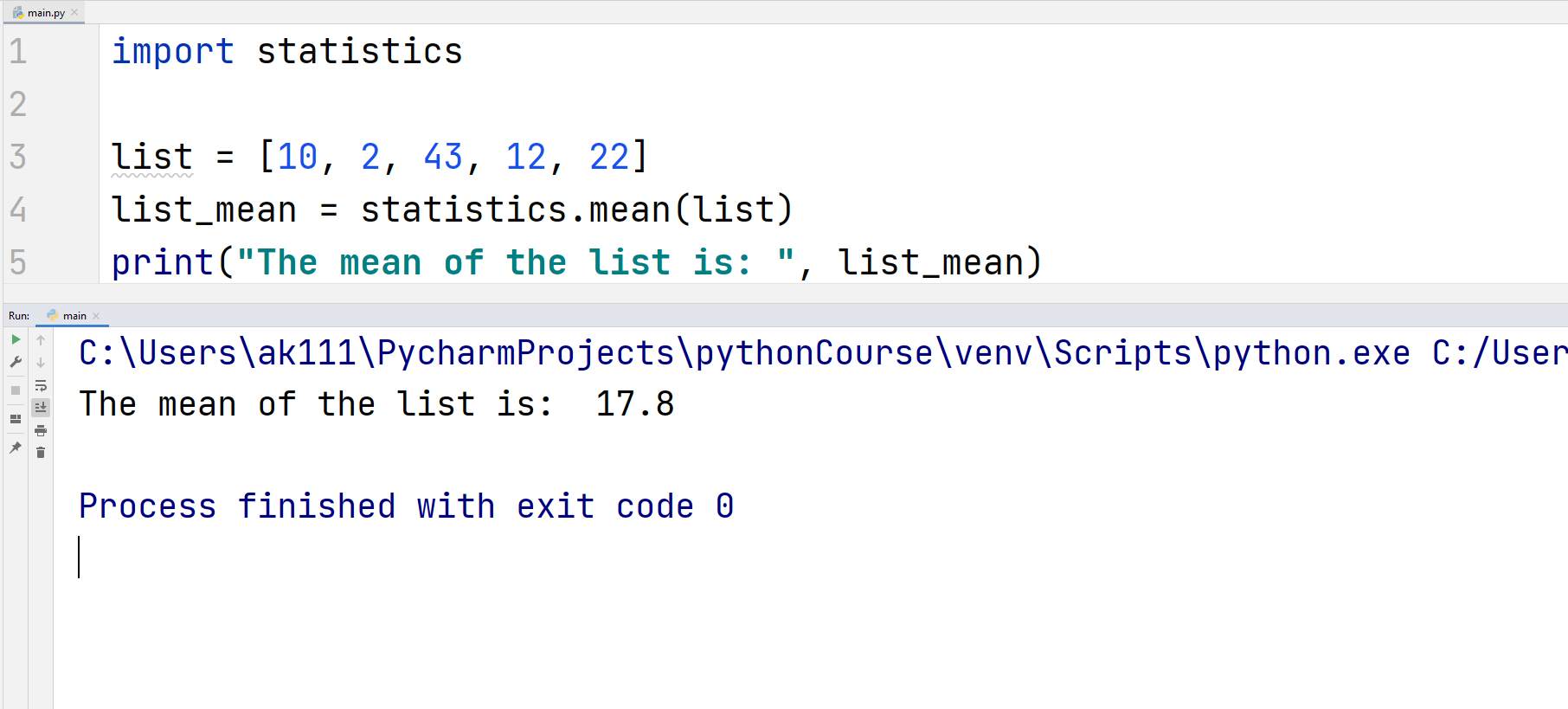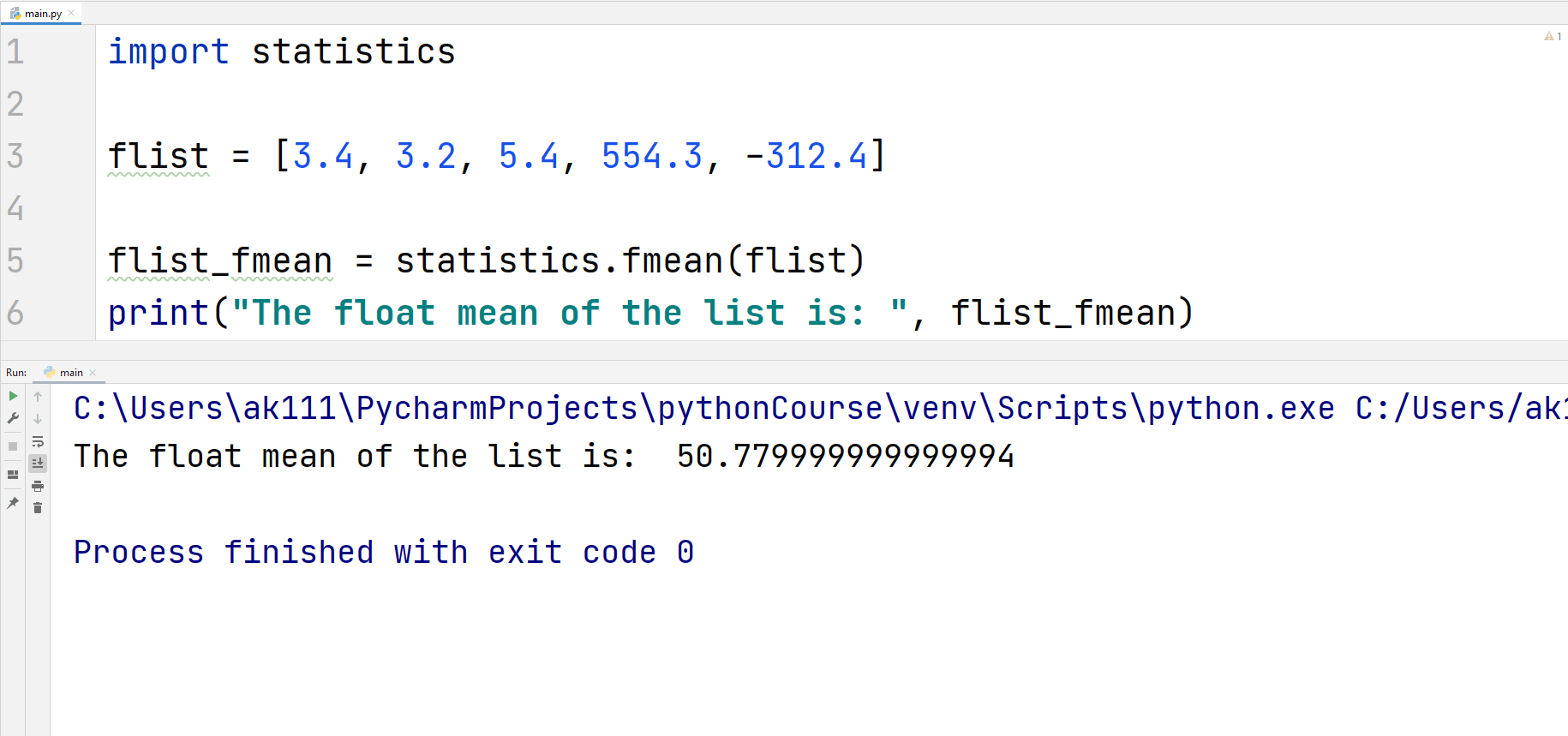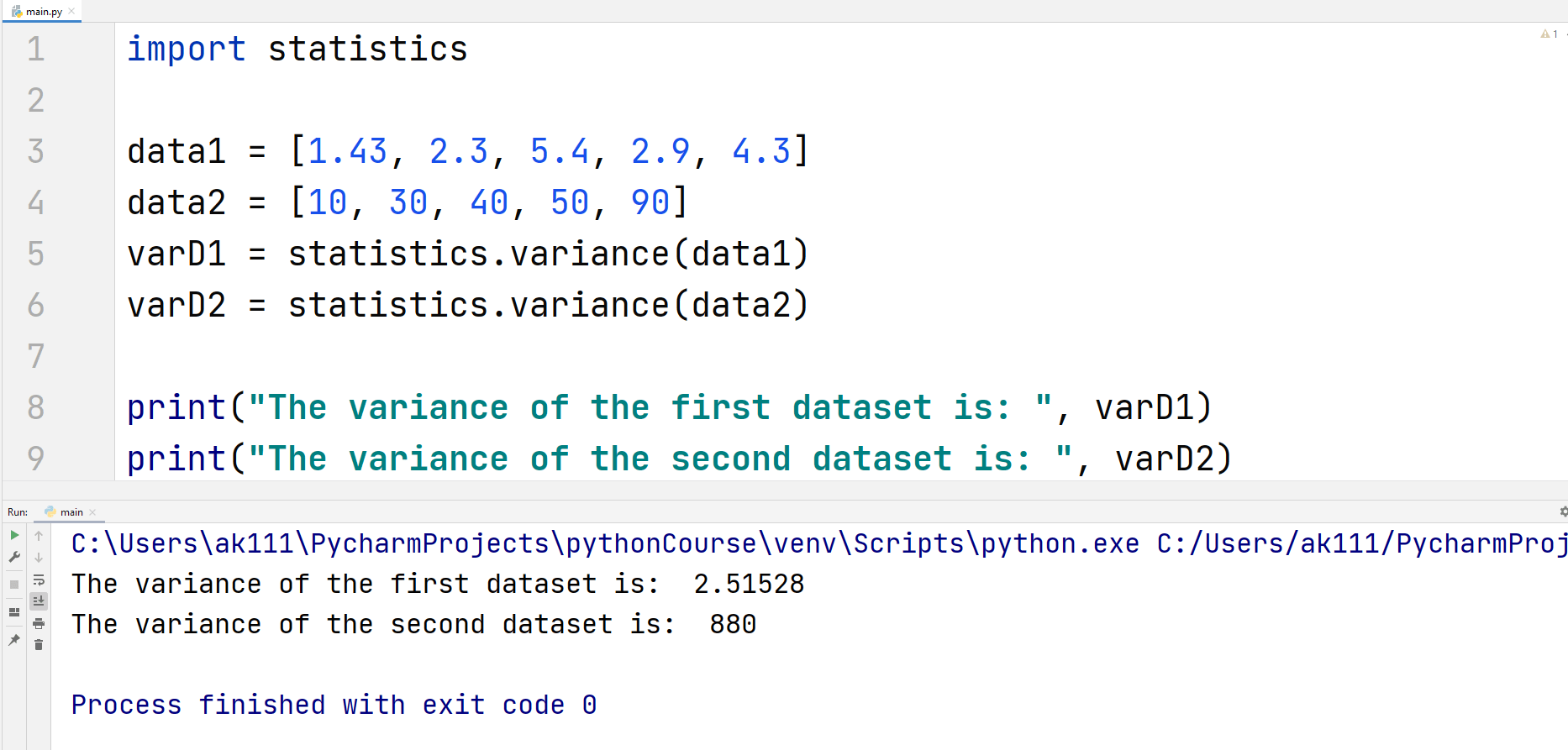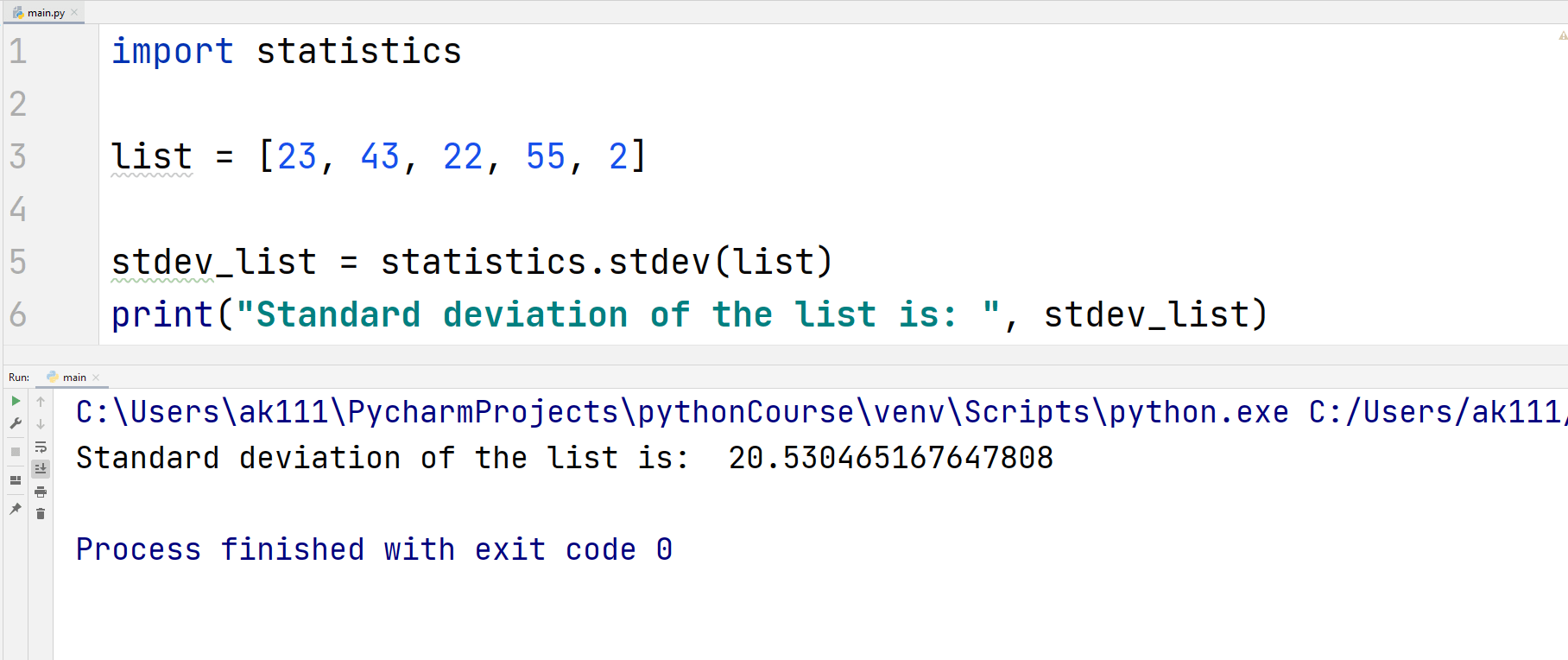# Python Statistics Module

## Python Statistic Module

The mathematics game is strong with Python. You get many functions related to computing mathematical operations built-in like finding the minimum number in a list.

The statistics module in Python just adds more to the mathematical capabilities of Python. You can, for example, calculate the median of numbers in a list without remembering or googling up what the formula to calculate the median is! Isn’t that great?

This along with libraries like Numpy, etc., is what makes Python a great tool for Statistics and Machine Learning.

## Must-Know Statistics Methods

Let’s cover the important functions of the statistics module in Python.

## mean() and fmean()

The mean() function returns the arithmetic mean of a data sample. The data sample can be an iterable or a sequence.

If you don’t already know, the mean is just the fancy name for the average of all data points, i.e. sum of all data points divided by the number of data points.

Let’s have an example of this function.

## ExampleA similar function to mean() is the fmean() function. fmean() converts the data points to a float integer and always returns a float value.

fmean() works faster than the mean() function.

## Example## median()

Median is the middle value of a numeric dataset. So, the median() function returns the middle value of a dataset.

## Example## variance() and stdev()

The variance() method returns the sample variance of the data passed into it. The returned value contains an iterable having at least two real numbers.

What does variance mean? If the value of variance is small, the data points are near to each other and vice versa. It is basically the degree of variation in a dataset.

Let’s have an example.

## ExampleAs you can see the second dataset data2 has a larger variance than data1 due to the noticeable bigger gap between data points.

The stdev() function calculates the standard deviation which is just equal to the square root of the variance. In a more definitive way, standard deviation is the measure of how much every data point, on average, is away from other data points.

Let’s have an example of standard deviation.

## ExampleThat was all for the most important statistics module functions in Python. Now, let’s see statistics functions that might come handy someday.

### Other Statistics Methods

 Function Name Function Description harmonic_mean() This function returns the central location of a dataset. median_high() Returns the high median of the dataset passed in the function.This is always the middle value if the number of data points is odd. And if the number of data points is even, this is equal to the larger value of the two middle values. median_low() Returns the low median of the dataset passed in the function.This is always the middle value if the number of data points is odd. And if the number of data points is even, this is equal to the smaller value of the two middle values. median_grouped() Returns the median of a grouped dataset. pstdev() Returns the population standard deviation of the dataset passed into it. pvariance() Returns the population variance of the dataset passed into it.